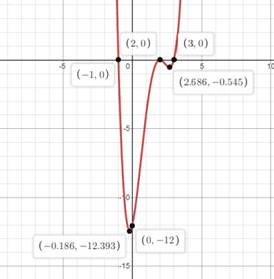# Tofind: The solution of the interval on x-axis in which curve lies under x-axis.### Precalculus: Mathematics for Calcu...

6th Edition
Stewart + 5 others
Publisher: Cengage Learning
ISBN: 9780840068071### Precalculus: Mathematics for Calcu...

6th Edition
Stewart + 5 others
Publisher: Cengage Learning
ISBN: 9780840068071

#### Solutions

Chapter 1.9, Problem 69E
To determine

## Tofind:The solution of the interval on x-axis in which curve lies under x-axis.

Expert Solution

The solution is interval on x-axis in which the curve lies under x-axis

x[1,3] .

### Explanation of Solution

Given: (x-2)2(x-3)(x+1)0

Concept used:

The lowest value or the roots is taken as minimum point where the solution exist.

The highest value or the roots is taken as maximum point where the solution exist.

The graph can predict the solution below x-axis.

Calculation:

Required:

(x-2)2(x-3)(x+1)0

Solve the graphically the inequality

1x3 or x[1,3] .

The solution is interval on x-axis in which the curve lies under x-axis.

x[1,3] .

Graph of (x-2)2(x-3)(x+1) :Hence, the solution is interval on x-axis in which the curve lies under x-axis

x[1,3] .

### Have a homework question?

Subscribe to bartleby learn! Ask subject matter experts 30 homework questions each month. Plus, you’ll have access to millions of step-by-step textbook answers!